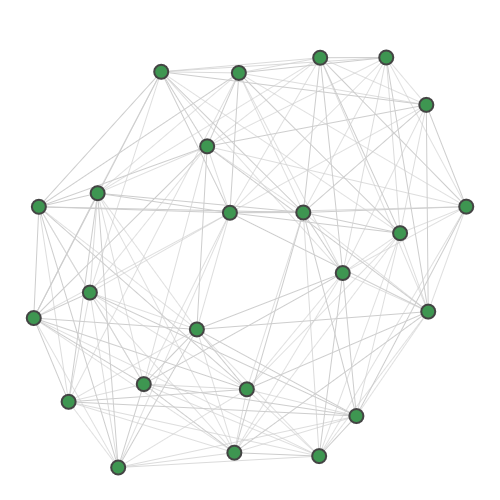# sf.apps.data.sample.TaceAs¶

class TaceAs[source]

Binding interaction graph for the TACE-AS complex .

Nodes in this graph correspond to pairs of atoms in a target protein and a pharmaceutical molecule. Edges in the graph are added if the distance between both pairs of atoms is very close to equal. Cliques in the graph correspond to possible docking configurations of protein and molecule, and the largest clique is the most stable configuration. There are multiple maximum-sized cliques of 8 nodes in this graph.

Graph:n_mean = 8
threshold = True
n_samples = 50000
modes = 24
n_mean = 8
threshold = True
 counts([axis]) Count number of photons or clicks.
counts(axis=1)

Count number of photons or clicks.

Counts number of photons/clicks in each sample (axis==1) or number of photons/clicks in each mode compounded over all samples (axis==0).

Parameters

axis (int) – axis to perform count

Returns

counts from samples

Return type

list

### Contents

Using Strawberry Fields

Development

API